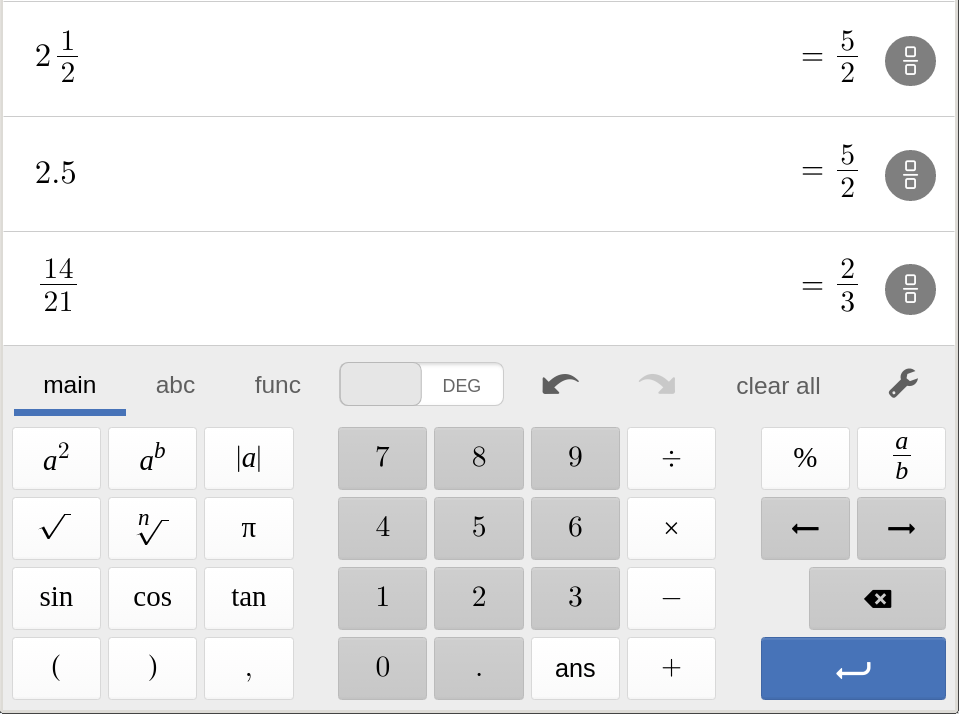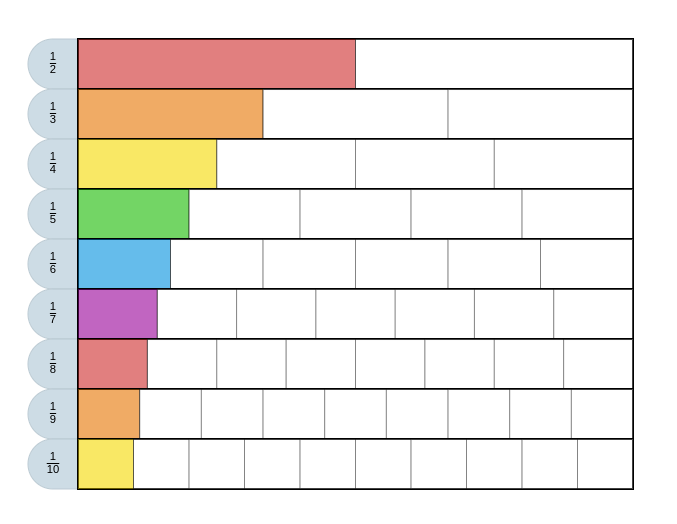## MTH212 Foundations of Elementary Math 2

### Fun with Fractions.

Notes on Fractions
Winter 2020

### Chapter 5: Integers and Fractions

#### § 5.2 Introduction to Fractions

Topics

• Fraction terminology
• "numerator"/"denominator"
• 0 < proper fraction < 1
• Improper fraction > 1
• Mixed number 2 1/2
• Fraction Concepts (Representations)
• Part of a whole area• Part of a whole group• Position on a number line• Division• Ratio• Equality of Fractions
Simplifying fractions
• Grouping with models• division with numerator and denominator

6/12=(2*3)/(2*6)=3/6

• factoring and the GCF
• Simplyfying Fractions with Calculators• Common Denominators
• LCM of denominators
• Signs: -2/3=(-2)/3=2/(-3)
since the quotient of dividing numbers of oposite sign is a negative number.
• Test for Equality
a/b=c/d if and only if ad=bc
• Inequality, Fraction comparisons• equal
4/12=7/21
• less than
1/3<1/2
• greater than
3/4>7/10 since 3*10 > 7*4
• Density of fractionsZoom in on the interval from 1/4 to 1/3• Mixed numbers
why 7/3 = 2 1/3
• Mental calculations
• Approximations